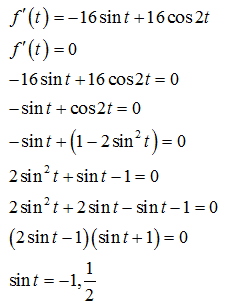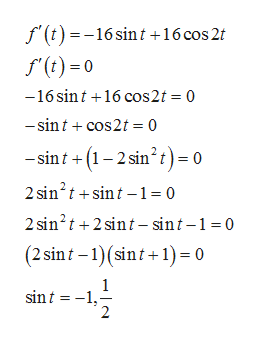# Find the absolute minimum and absolute maximum values of f on the given interval2sin2t after differentiating in order to be able to factor the derivative.Hint: You will need to use the identity cos 2t = 1 -= 16 cos t + 8 sin 2t,f(t)[0, π/2]

Questionhelp_outlineImage TranscriptioncloseFind the absolute minimum and absolute maximum values of f on the given interval 2sin2t after differentiating in order to be able to factor the derivative. Hint: You will need to use the identity cos 2t = 1 - = 16 cos t + 8 sin 2t, f(t) [0, π/2] fullscreen
check_circleExpert Solution
Step 1

Given function is

Step 2

To find critical point of f(t), we solve f'(t)=0help_outlineImage Transcriptionclosef(t)=-16 sin t +16 cos 2 f(t)0 -16 sint 16 cos2t 0 -sintcos2t 0 sint(12 sin2t)= 0 2 sin2tsint-1= 0 2 sin2t2 sin t - sint-1 0 (2 sint 1(sint+1)= 0 1 sint 1 2 fullscreen
Step 3

Since t lies in [0,pi/2], so only critical ...

### Want to see the full answer?

See Solution

#### Want to see this answer and more?

Solutions are written by subject experts who are available 24/7. Questions are typically answered within 1 hour*

See Solution
*Response times may vary by subject and question
Tagged in

### Derivative